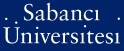# Introduction to stochastic programming and its applications to finance

Şimşek, Koray Deniz (2012) Introduction to stochastic programming and its applications to finance. In: Fabozzi, Frank J., (ed.) Encyclopedia of Financial Models. Wiley, Hoboken, NJ, pp. 123-135. ISBN 9781118006733 ; 1118006739PDF (Final Proof PDF) - Repository staff only - Requires a PDF viewer such as GSview, Xpdf or Adobe Acrobat Reader289Kb

## Abstract

Mathematical programming is one of a number of operations research techniques that employs mathematical optimization models to assist in decision making.Mathematical programming includes linear programming, integer programming, mixed-integer programming, nonlinear programming, stochastic programming, and goal programming. Mathematical programming models allow the decision maker to identify the “best” solution. This is in contrast to other mathematical tools that are in the arsenal of decision makers such as statistical models (which tell the decision maker what occurred in the past), forecasting models (which tell the decision maker what might happen in the future), and simulation models (which tell the decision maker what will happen under different conditions). The mean-variance model for portfolio selection as formulated by Markowitz is an example of an application of one type of mathematical programming (quadratic programming). However, in formulating optimization models in many applications in finance, decision makers need to take into consideration the uncertainty about the model’s parameters and the multiperiod nature of the problem faced. To deal with these situations, the technique of stochastic programming is employed.

Item Type: Book Section / Chapter H Social Sciences > HG Finance 20588 Koray Deniz Şimşek 06 Dec 2012 14:43 31 Jul 2019 15:39

Repository Staff Only: item control page